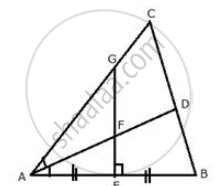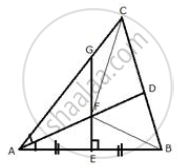# The Given Figure Shows a Triangle Abc in Which Ad Bisects Angle Bac. Eg is Perpendicular Bisector of Side Ab Which Intersects Ad at Point F. Prove That: - Mathematics

Sum

The given figure shows a triangle ABC in which AD bisects angle BAC. EG is perpendicular bisector of side AB which intersects AD at point F.
Prove that:F is equidistant from A and B.

#### SolutionConstruction: Join FB and FC
Proof: In ΔAFE and ΔFBE,
AE = EB (E is the mid-point of AB)
∠FEA = ∠FEB (Each = 90°)
FE = FE (Common)
∴ By side Angle side criterion of congruence,
ΔAFE ≅ ΔFBE (SAS Postulate)
The corresponding parts of the congruent triangles are congruent.
∴ AF = FB (CPCT)
Hence, F is equidistant from A and B.

Concept: Theorems Based on Loci
Is there an error in this question or solution?

#### APPEARS IN

Selina Concise Maths Class 10 ICSE
Chapter 16 Loci (Locus and Its Constructions)
Exercise 16 (A) | Q 11.1 | Page 238